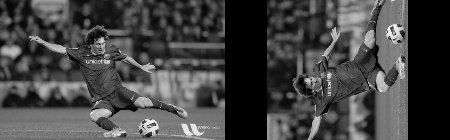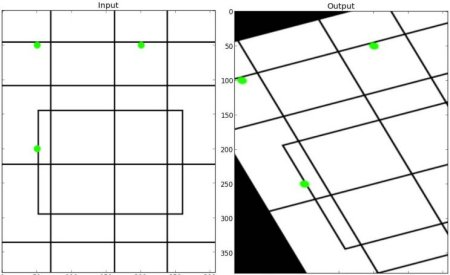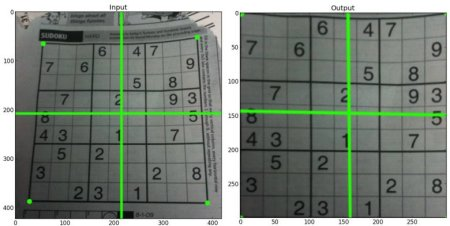OpenCV  4.0.1 Open Source Computer Vision
Geometric Transformations of Images

## Goals

• Learn to apply different geometric transformation to images like translation, rotation, affine transformation etc.
• You will see these functions: cv.getPerspectiveTransform

## Transformations

OpenCV provides two transformation functions, cv.warpAffine and cv.warpPerspective, with which you can have all kinds of transformations. cv.warpAffine takes a 2x3 transformation matrix while cv.warpPerspective takes a 3x3 transformation matrix as input.

### Scaling

Scaling is just resizing of the image. OpenCV comes with a function cv.resize() for this purpose. The size of the image can be specified manually, or you can specify the scaling factor. Different interpolation methods are used. Preferable interpolation methods are cv.INTER_AREA for shrinking and cv.INTER_CUBIC (slow) & cv.INTER_LINEAR for zooming. By default, interpolation method used is cv.INTER_LINEAR for all resizing purposes. You can resize an input image either of following methods:

import numpy as np
import cv2 as cv
res = cv.resize(img,None,fx=2, fy=2, interpolation = cv.INTER_CUBIC)
#OR
height, width = img.shape[:2]
res = cv.resize(img,(2*width, 2*height), interpolation = cv.INTER_CUBIC)

### Translation

Translation is the shifting of object's location. If you know the shift in (x,y) direction, let it be $$(t_x,t_y)$$, you can create the transformation matrix $$\textbf{M}$$ as follows:

$M = \begin{bmatrix} 1 & 0 & t_x \\ 0 & 1 & t_y \end{bmatrix}$

You can take make it into a Numpy array of type np.float32 and pass it into cv.warpAffine() function. See below example for a shift of (100,50):

import numpy as np
import cv2 as cv
rows,cols = img.shape
M = np.float32([[1,0,100],[0,1,50]])
dst = cv.warpAffine(img,M,(cols,rows))
cv.imshow('img',dst)

warning

Third argument of the cv.warpAffine() function is the size of the output image, which should be in the form of **(width, height)**. Remember width = number of columns, and height = number of rows.

See the result below:image

### Rotation

Rotation of an image for an angle $$\theta$$ is achieved by the transformation matrix of the form

$M = \begin{bmatrix} cos\theta & -sin\theta \\ sin\theta & cos\theta \end{bmatrix}$

But OpenCV provides scaled rotation with adjustable center of rotation so that you can rotate at any location you prefer. Modified transformation matrix is given by

$\begin{bmatrix} \alpha & \beta & (1- \alpha ) \cdot center.x - \beta \cdot center.y \\ - \beta & \alpha & \beta \cdot center.x + (1- \alpha ) \cdot center.y \end{bmatrix}$

where:

$\begin{array}{l} \alpha = scale \cdot \cos \theta , \\ \beta = scale \cdot \sin \theta \end{array}$

To find this transformation matrix, OpenCV provides a function, cv.getRotationMatrix2D. Check below example which rotates the image by 90 degree with respect to center without any scaling.

rows,cols = img.shape
# cols-1 and rows-1 are the coordinate limits.
M = cv.getRotationMatrix2D(((cols-1)/2.0,(rows-1)/2.0),90,1)
dst = cv.warpAffine(img,M,(cols,rows))

See the result:image

### Affine Transformation

In affine transformation, all parallel lines in the original image will still be parallel in the output image. To find the transformation matrix, we need three points from input image and their corresponding locations in output image. Then cv.getAffineTransform will create a 2x3 matrix which is to be passed to cv.warpAffine.

Check below example, and also look at the points I selected (which are marked in Green color):

rows,cols,ch = img.shape
pts1 = np.float32([[50,50],[200,50],[50,200]])
pts2 = np.float32([[10,100],[200,50],[100,250]])
M = cv.getAffineTransform(pts1,pts2)
dst = cv.warpAffine(img,M,(cols,rows))
plt.subplot(121),plt.imshow(img),plt.title('Input')
plt.subplot(122),plt.imshow(dst),plt.title('Output')
plt.show()

See the result:image

### Perspective Transformation

For perspective transformation, you need a 3x3 transformation matrix. Straight lines will remain straight even after the transformation. To find this transformation matrix, you need 4 points on the input image and corresponding points on the output image. Among these 4 points, 3 of them should not be collinear. Then transformation matrix can be found by the function cv.getPerspectiveTransform. Then apply cv.warpPerspective with this 3x3 transformation matrix.

See the code below:

rows,cols,ch = img.shape
pts1 = np.float32([[56,65],[368,52],[28,387],[389,390]])
pts2 = np.float32([[0,0],[300,0],[0,300],[300,300]])
dst = cv.warpPerspective(img,M,(300,300))
plt.subplot(121),plt.imshow(img),plt.title('Input')
plt.subplot(122),plt.imshow(dst),plt.title('Output')
plt.show()

Result:image